Science Journal of Applied Mathematics and Statistics
Volume 4, Issue 5, October 2016, Pages: 242-248

Quantile Regression Model for Measurement of Equity Portfolio Risk a Case Study of Nairobi Securities Exchange

Kinyua Mark Njega1, Joseph Kyalo Mung’atu2

1Applied Statistics, Department of Statistics and Actuarial Science, Jomo Kenyatta University of Agriculture and Technology, Nairobi, Kenya

2Statistics, Department of Statistics and Actuarial Science, Jomo Kenyatta University of Agriculture and Technology, Nairobi, Kenya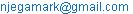(K. M. Njega)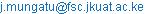(J. M. Kyalo)

Kinyua Mark Njega, Joseph Kyalo Mung’atu. Quantile Regression Model for Measurement of Equity Portfolio Risk a Case Study of Nairobi Securities Exchange. Science Journal of Applied Mathematics and Statistics. Vol. 4, No. 5, 2016, pp. 242-248. doi: 10.11648/j.sjams.20160405.18

Received: September 9, 2016; Accepted: September 21, 2016; Published: October 9, 2016

Abstract: Quantile regression provides a method of estimating quantiles from a conditional distribution density. It is achieves this by minimizing asymmetrically weighted sum of absolute errors thus partitioning the conditional distribution into quantiles. Lower conditional quantiles are of interest in estimation of Value-at-Risk because they indicate downward movement of financial returns. Current risk measurement methods do not effectively estimate the VaR since they make assumptions in the distribution tails. Financial data is sampled frequently leading to a heavier tailed distribution compared to a normal and student t distribution. A remedy to this is to use a method that does not make assumptions in the tail distribution of financial returns. Little research has been done on the usage of quantile regression in the estimation of portfolio risk in the Nairobi Securities Exchange. The main aim of this study was to model the portfolio risk as a lower conditional quantile, compare the performance of this model to the existing risk measurement methods and to predict the Value-at-Risk. This study presents summary of key findings and conclusion drawn from the study. From the fitted conditional quantile GARCH model 62.4% of VaR can be explained by past standard deviation and absolute residual of NSE 20 share index optimal portfolio returns. The fitted model had less proportion of failure of 7.65% compared to commonly used VaR models.

Keywords: Quantile Regression, GARCH, Value-at-Risk

1. Introduction

1.1. Background of the Study

The establishment of Nairobi Securities Exchange (NSE) dates back to 1954 when it was registered under the Societies Act as a voluntary association of stock brokers. According to Capital Market Authority of Kenya (CMA), NSE is an approved institution in Kenya that is charged with the responsibility of developing securities markets and regulating trading activities. It has an automated trading system that provides a platform for trading of financial products. In 2007, a Wide Area Network (WAN) was established in the NSE enabling stock brokers to carry out trading activities from their offices. Like any other automated securities market, financial asset prices fluctuate from time to time, which forms the basis of gain or loss by investors. Maximizing profit and minimizing loss is the main objective of any investor/shareholder in a stock market hence risk management is essential in trading of financial assets in a stock market.

Capital Market Authority of Kenya in 2010 noted a rising trend in equity stock trading at the NSE. This hasprompted the development of risk management strategies by financial researchers so as to sustain the ever increasing investment appetite among investors (Rao and Tata, 2012) . Fischer and Jordan (2003)  noted that the area of technical risk and return analysis need to be given some considerations. Financial researches make use past information as a source of insights to quantify risk. According to Research Foundation of CFA institute (2009), risk management is an act as well as a science that quantifies risk through risk measurement and also provides a concrete understanding of the nature of risk.

According to (Rao and Tata, 2012)  Value-at-Risk (VaR) is the percentage loss in market value over a given time horizon that is exceeding with a given a probability. In addition, Value-at-Risk is a conditional quantile of the return series and estimation of the VaR is intimately linked to quantile regression. The lower tails of the return series distribution help in the assessment of the loss associated with the downward movement of the share prices. Quantile regression as introduced by (Koenker and Basset 1978)  provides a way of estimating the conditional quantile without making distribution assumptions.

1.2. Statement of the Problem

Investment involves allocating funds with an expectation of earning profits in future. However, states of nature are the ones that determine whether there will be gains or losses. This is beyond human cognition and the best way to undergo this is by managing risk. Loss that is associated with the downward movement of asset prices forms the basis of risk measurement (VaR). Most existing methods of calculating the VaR assume that the tail distribution of the returns follow a certain distribution. This is not the case in financial returns as data is sampled frequently leading to a heavy tailed distribution. In addition, making assumption in the tails of a distribution does not provide a way of capturing extreme shocks such as those experienced in financial data. Quantile Regression as proposed by (Koenker and Basset 1978)  provides a better way of capturing the outliers in financial time series. This is due to the fact that it models the relationship of the covariates effect on the conditional quantile of the response variable without making assumption of the conditional distribution. Additionally, little research has been made on quantile regression in the estimation of the portfolio Value-at-Risk in the Nairobi Securities Exchange. The use of a method that incorporates extreme shocks (Quantile Regression) is a better way of estimating the minimum value that an investor is willing to loss given a certain probability (VaR).

1.3. Justification of the Study

The findings of this study are of importance to the participants of the NSE. These include the equity stock investors, stock brokers, market regulators, policy makers and other parties who recognize the significance of quantifying risk as a risk management strategy. Investors and financial advisers are placed in a better position to measure risk and limit trading activities. This consequently helps in minimizing risk and maximizes profits by investing in a combination of securities. In addition to this, financial activities will increase leading to a competitive trading environment which will contribute to the economy growth. Through a competitive environment, investors and anyone willing to invest in the stock exchange is able to access the transparent information on all stocks. Financial researchers have an opportunity onidentification of researchproblemas well as solutions associated with investment in the Securities exchange.

1.4. Objectives

1.4.1. General Objective

This study sort to estimate portfolio risk measures using quantile regression of an optimal portfolio of equities chosen from listed companies trading at the Nairobi Securities Exchange 20 share index for the period of July 2010 - June 2016.

1.4.2. Specific Objectives

a)  To determine an optimal portfolio from the listed companies in NSE 20 share index.

b)  To estimate the Quantile GARCH based Value-at-Risk of an optimal equity portfolio from Nairobi Securities Exchange.

c)  To evaluate the back test forecast performance of the quantile GARCH based model.

2. Review of the Previous Studies

The application of conditional quantile estimation for VaR has been extensively applied by most financial researchers. There is accumulated evidence among financial practitioners that this data exhibits negative skewness and excess positive kurtosis as compared to the parametric models assumptions. Thus parametric model do not perform well as compared to non parametric and semi parametric. Non parametric conditional quantile estimations have also been applied but they suffer from the inability to accommodate more than two covariates (Rao and Tata 2012) . It is due to this reason that researcher embarked on coming up with robust methods of estimating the Value-at-Risk. A remedy is to use the semi parametric methods such as quantile regression as introduced by (Koenker and Bassett 1978) . (Kraus and Czado 2015)  Stresses this by stating the quantile regression has gained importance in statistical modeling and financial applications.

Estimation of conditional quantiles and variances in the analysis of financial time series is essential in risk management as noted by (Chen, 2009) . According to (Bassett et al. 2004) , conditional quantile distribution information forms the basis of estimating Value-at-Risk, expected shortfall and limited expected loss which help in quantifying risk. VaR is a single value and is easy to interpret has been a regulatory concept used in most of the financial institution since its introduction in October 2004.

GARCH models as introduced by (Bollerslev, 1986)  have successfully been used in modeling of financial data since they are able to capture long influence of past shocks. Based on this model, (Engle and Manganelli 2004) , suggested a nonlinear dynamic quantile auto regression on the evolution of time varying standard deviation. (Xiao and Koenkar 2009)  Argue that this model is complicated to estimate and existing nonlinear quantile estimation methods cannot be directly applicable in his model. (Rossi and Harvey, 2009)  Applied Kalman filter in the estimation of the dynamic quantiles based on CAViaR model. (Xiao and Koenkar 2009) , suggested a two step approach in the estimation of the conditional quantile based on a GARCH model.

This research project used the two step estimation procedure using GARCH model as suggested by (Xiao and Koenkar 2009) . (Bollerslev, 1986)  GARCH model enables one to model conditional variance on past squared errors and previous conditional variance. However (Duffie, 1997) noted that the maximum likelihood estimation of the (Bollerslev, 1986)  model had a disadvantage of overshooting extreme returns. Thus a modified GARCH as suggested by (Taylor, 2007)  is used in the estimation procedure. The first step involves estimation of a sieve quantile auto regressive process of the time varying standard deviation. Second step involve carrying out quantile regression of the past time varying shocks on the estimated standard deviation and past absolute innovations.

(Machuke, 2014)  in their study on measurement of stock return risk in NSE considered two of the best performing stocks. The stocks considered were Bamburi and BAT which are from the same industry thus unsystematic risk was not taken care of. They further concluded that risk metrics did not effectively estimate the Value-at-Risk and recommended the usage of semi parametric and non parametric methods. This project worked towards incorporating the unsystematic risk through creating a portfolio and using the quantile regression which is a semi parametric method.

3. Research Methodology

In this section, we discuss the specification of the quantile GARCH based model, estimation procedure using two stage method and the goodness of fit of the model. Lastly, the model performance measures by use of back test forecast.

3.1. Specification of the Model

Portfolio returns are weighted averages of the individual individual assets that form a portfolio.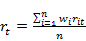(1)

where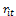are the simple returns of the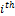,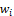are the weigths of individual assets,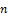is the number of companies/assets in the portfolio and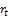is the portfolio return.

Considering a series of portfolio returns in (1)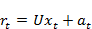(2)

Whereis the portfolio return series,is the mean equation of the series and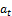is the innovations/shocks of the return series inequation (2).

Conditional heteroscedasticity models mainly focus on the shocks of the process. A linear GARCH process as suggested by (Taylor, 2007)  is considered in this study. An advantage of this model is that it is less sensitive to extreme shocks.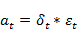(3)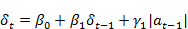(4)

In equation (3),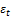is an independently and identically standard normal error. In equation (4),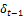and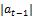is the past time varying standard deviation and absolute residuals of the return series.

3.2. Estimation of Lower Conditional Quantile

Quantile regression as suggested by (Koenker and Bassett 1978)  provides a robust way of estimating a conditional quantile of a probability distribution without making distribution assumptions. However, according to (Xiao and Koenkar 2009) , quantile GARCH based model is highly nonlinear due to the dependencies of the latent and the unknown parameters. The authors further suggest that an estimation method that fully incorporates the global dependencies is the best strategy. To circumvent this problem a two step estimation procedure was used for the following restricted optimization problem in Equation (5). This quantile regression problem seeks to emphasize on the global dependency.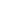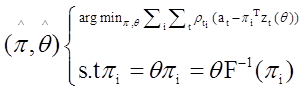(5)

The study aimed at computing the Value-at-Risk as a lower conditional quantile of the return series.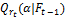denotes the conditional quantile while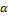being the quantile of interest and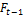being the past information up to time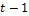. Under weak regularity condition as noted by (Xiao, 2009), the conditional quantile estimate is given by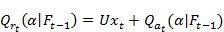(6)

Focusing on the conditional quantile of the shocks of the return series in Equation (5) gives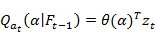(7)

Whereis a vector of unknown quantile regression parameters and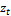contains latent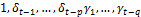. Thus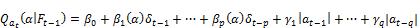(8)

3.3. Two Step Estimation Procedure

The first step involves incorporation of the global dependence of the latent and the unknown parameters via minimum distance method to construct a global estimate of the conditional scale parameter. That is obtained by a sieve quantile auto regression of the time varying standard deviation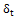at a specific quantile of Equation (3). Second step involves using results of the first step to estimate local conditional quantile as in Equation (7). Using the estimates of the time varying quantile auto regression we use the multiplicative standard normal error to get the innovations/shocks of the return series. A quantile regression of the new series of shocks on past estimated time varying standard deviationand past absolute innovation/shocksboth up to time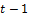.

3.4. Goodness of Fit of the Model

Rank-Inverse Score is a test that is designed to test the goodness of fit of the quantile regression estimates of a sparse distributed times series. According to Koenker and Machado (1999), Regression rank-score is a process that establishes the link between linear rank statistics and regression quantiles. Quantile regression is a linear programming problem that results to a dual problem which is used to formulate the regression quantiles. Koenker and Machado (1999) generalized the duality of ranks and quantile to linear programming models and suggested a process that establishes a link between linear rank statistics and regression quantiles commonly known as regression rank score. Rank-inverse score is thus achieved by integrating the regression rank score to an appropriate signed measure on (0, 1). Additionally, it helps in testing the significance of the QR coefficients. Pseudo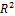is used to measure the goodness of fit of a particular quantile rather than a global goodness of fit over entire conditional distribution.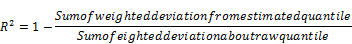Sum of weighted deviation from the estimated quantile is the solution given by the optimization problem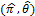while sum of deviation about raw quantile is that value that partitions the return distribution into equal portions.

3.5. Back Test of the VaR Model

According to (Nieppola, 2009)  VaR models are useful if they can accurately predict the future risk. To evaluate the performance of the VaR model appropriate evaluation methods must be used. This means Value-at- Risk model is only as good as its back test. Additionally the financial researcher states that computation of VaR is adequate after reporting back test

Value-at-Risk is an out of sample concept therefore VaR models are evaluated using a systematic procedure that compares the actual losses and predicted conditional quantiles. (Kupiec, 1995)  suggested a proportion of failures test that seeks to statistically find out if the frequency of exceptions is in line with the confidence interval. Kupiec test aims at computing coverage rate (proportion of realized quantiles that fall below the predicted quantiles). Coverage rates go hand in hand with the dependencies of the predicted conditional quantiles. (Christofferssen, 2004)suggested a conditional coverage test to evaluate the dependencies.

3.6. Description of the Data

In this study, data was obtained from Nairobi Securities Exchange data center as the daily closing prices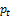of all companies listed for a period of July 2010 to June 2016. This period of study was guided by the Kenyan Government financial year. The study used a sample of 25 companies listed in the NSE 20 share index between January 2000 and December 2015. A review of the listed companies is done over a period of 15 years and decisions are made on whether to include or drop the company from the index. This is the main reason as to why the index was used as guidance to the sample selection. The other reason as to why the other companies were excluded is because of the limited number of observations and had inconsistent trading during the study period. Simple returnswere calculated as difference of natural logarithm of consecutive closing equity prices of listed companies.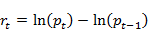(9)

Whereis the daily closing equity price at time t and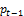is the daily opening equity price Equation (8).

4. Results and Discussion

An optimal portfolio was selected based on the returns of individual listed companies. Portfolio returns were calculated forming the basis of estimation of the conditional quantile. Package fportfolio in R software (R Core Team 2015)  was used to select an optimal portfolio. Package Performance Analytics was used to compute portfolio returns as well as plotting and package Quant Reg was used to find the conditional quantile function.

4.1. Optimal Portfolio Selection

An optimal portfolio was constructed using the Capital Asset Pricing Model (CAPM) where companies with negative correlation and co variances were picked. A total of seven companies were selected as in table 1.

Table 1. List of companies making optimal portfolio.

 Symbol NSE Equity Security Industry SCOM Safaricom Limited Telecommunication & technology BAT British American Tobacco Limited Manufacturing & Allied KUKZ Kakuzi Limited Agriculture SASN Sasini Limited Agriculture SGL Standard Group Limited Commercial & Services JUB Jubille Holdings Limited Insurance OCH Olympia Capital Holdings Limited Investment

It is clear from the selected companies that they are from different sectors/industries indicating it is a diversified portfolio.This is inline with the finding of Grinblatt and Keloharju (2001)  who noted that diversification eliminates the unsystematic risks and lowers the total market risk which can not be diversified away.

4.2. Portfolio Returns

Portfolio returns were split into two that is the in sample data (1450) and out of sample data (290). returns. Table 2 presents a summary statistics of the portfolio returns based on training dataset.

Table 2. Summary statistics of portfolio returns.

 Mean 0.22164948 Standard Deviation 2.66342653 Minimum -6.93394951 Maxmum 6.45650550 Kurtosis -0.0326 Skewness 2.492755 Jarque-Bera 236.65 Probability 2.2*10-16

The mean daily returns of the optimal portfolio returns were 0.222% and daily standard deviation of 2.66%. The portfolio returns displayed a negative skewness of -0.033 and excess positive kurtosis of 2.493. Additionally on performing a test for normality (Jarque & Bera, 1980) a p-value of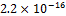was reported. This value is less than 0.05 level of significance leading to the rejection of the null hypothesis that the distribution of the returns are normally distributed. The conclusion is that the distribution is non normal and exhibits fat tails (leptokurtic). This is inline with most of the findings of the financial researchers that financial data distribution is heavily tailed.It is clear from the test of normality that the distribution of the portfolio returns are heavily tailed and therefore, a method that does not make assumption on the lower tails was used. A quantile GARCH based model was used to estimate the lower conditional quantile.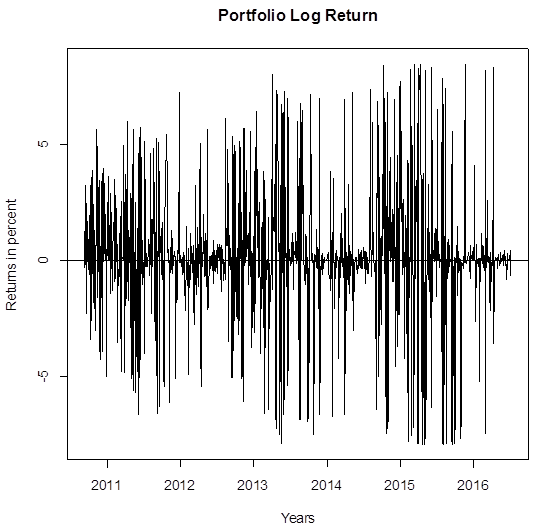Figure 1. Plot of portfolio returns against years.

4.3. GARCH Model

The underlying GARCH model for the portfolio returns was estimated before developing the Quantile GARCH based model. Figure 1 show a plot shows volatility clustering since high volatility parts tend to be followed by high volatility parts hence presence of ARCH effects in the data.

Table 3. Test for conditional heteroscedasticity.

 Box-Ljung Test Chi-square Df P-value 236.65 12 2.2×10-16

Table 3 shows results of Box Ljung test on the residuals of the returns series. A p-value ofwas reported leading the rejection of the null hypothesis that there are no ARCH effects.

Table 4. GARCH parameter estimates.

 Estimate Std. Error P-value mu 0.16460 0.06544 0.011891 omega 0.21586 0.06466 0.000843 alpha 1 0.0733 0.01266 0.00000 beta 1 0.89598 0.01896 0.00000

Table 4 shows that all parameters are significant at 0.05 level of significance hence a GARCH (1,1) model is the best in describing the conditional heteroscedasticity.

4.4. Quantile GARCH Based Model at 0.05 Quantile

Table 5. Quantile GARCH regression estimates.

 Quantile Latent Coef StdErr. P value 95% conf. Interval 0.05 Constant -1.958 0.654 0.02 -2.36, 0.095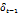0.059 0.318 0.0001 -1.13,0.062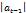0.009 1.536 0.0000 -1.79,0.0091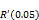=0.6236

Conditional quantile models were estimated that is at 0.05 quantile and the model denoted as QGARCH 1. The parameters of the model were as follows.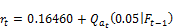(10)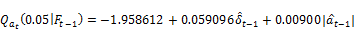(11)

4.5. Goodness of Fit

A measure of how well the 0.05 quantile GARCH based model over the entire conditional distribution (Equations (10) and (11)),of 0.6236 was reported. This value is high indicating that the 62.4% of the dependent variable (conditional quantile) can be explained by the independent variables (past time varying standard deviation and past absolute innovations).

4.6. Comparison with Other Risk Metrics Model

Value-at-risk is a conditional quantile and it is an out-of-sample concept. Out of sample data of 290 portfolio returns was used in the model evaluation performance. As a measure of performance coverage ratios were used to compare models. Coverage ratios were carried out as follows at each one step ahead t+1, data up to time t was used to forecast the next period conditional quantiles.

The comparison was done with the Gaussian GARCH VaR model and students t test with 4 degrees of freedom VaR model. Results for unconditional coverage (Kupiec test) and conditional coverage (Christoffersen test) were as follows;

Table 6. Unconditional coverage rates (Kupiec test).

 QGARCH Students-t VaR Normal VaR Coverage rate 0.0759 0.113 0.0962 Test statistics 3.6049 4.309 4.486 P-Value 0.0576 0.5115 0.0341 Decision Correct exceendacies Correct exceendacies Incorrect exceendacies

Table 7. Conditional coverage rates (Christoffersen test).

 QGARCH Students-t VaR Normal VaR Coverage rate 0.0759 0.113 0.0962 Test statistics 3.9748 4.041 5.006 P-Value 0.137 0.133 0.0294 Decision Correct dependencies Correct dependencies Incorrect dependencies

From the table 4 and 5, the QGARCH and students-t VaR models had a p-value of more than 0.05 meaning the models are able to adjust to unexpected changes in volatility. However the quantile GARCH based model performed better normal VaR did not perform well as compared to the student’s t distribution VaR and the Quantile GARCH based model. This is simply because the latter models take into account the fat tailed behavior of the log returns. On comparing the quantile GARCH based model and students t VaR, the fitted model perform better because it had lower proportion of failure of 7.65% compared to 11.3% of student t VaR model.

5. Conclusion and Recommendations

5.1. Conclusion

This chapter presents summary of key findings and conclusion drawn from the study. From the fitted conditional quantile GARCH model 62.4% of VaR can be explained by past standard deviation and absolute residual of NSE 20 share index optimal portfolio returns. The fitted model had less proportion of failure of 7.65% compared to commonly used VaR models.

5.2. Recommendations

The semi parametric approaches such as quantile regression are recommended for assessing the lower tails of a financial return distribution. Generalized Autoregressive Conditional Heteroscadasticity is useful for modeling non constant variance/standard deviation of optimal portfolio returns.

5.3. Further Research

Other GARCH models can be considered for the portfolioreturns such as Exponential GARCH, Threshold GARCH and CHARMA model.

References

1. Bassett, G. W., R. Koenker and G. Kordas (2004), ‘Pessimistic portfolio allocation and choquet expected utility’, Journal of financial econometrics 2(4), 477–492.
2. Bollerslev, T. (1986), ‘Generalized autoregressive conditional heteroskedasticity’, Journal of econometrics 31(3), 307–327.
3. Chen, X. a. (2009). Copula-based nonlinear quantileautoregression. The Econometrics Journal, 12, S50--S67.
4. Christofferssen, P. and P. Pelletier (2004), ‘Backtesting value-at-risk: A duration-based approach’, Journal of Empirical Finance 2, 84–108.
5. Engle, R. F. and S. Manganelli (2004), ‘Caviar: Conditional autoregressive value at risk by regression quantiles’, Journal of Business & Economic Statistics 22(4), 367–381.
6. Fischer, D.E. and R. J. Jordan (2003), Security Analysis and Portfolio Management,New Delphi, Prentice Hall of India Private Limited.
7. Grinblatt, Mark and Matti Keloharju (2001), ‘What makes investors trade?’, The Journal of Finance 56(2), 589–616.
8. Hsu, Ya-Hui (2010), Applications of quantile regression to estimation and detection of some tail characteristics, PhD thesis, University of Illinois at Urbana-Champaign.
9. Koenker, R. W. and G. Bassett (1978), ‘Regression quantiles’, Econometrica: journal of the Econometric Society 46, 33–50.
10. Kraus, D. and C. Czado (2015), ‘D-vine copula based quantile regression’, arXiv preprint arXiv:1510.04161.
11. Machuke, G., P. N. Mwita and J. M. Kihoro (2014), ‘Measuring financial risk in stock returns: A case study of nairobi securities exchange’, International Journal of Sc 3(4).
12. Kraus, D. a. (2015). D-vine copula based quantile regression. arXiv preprint arXiv:1510.04161.
13. Kupiec, P., "Techniques for Verifying the Accuracy of Risk Management Models", Journal of Derivatives 3 (1995), pp. 72-84.
14. Nieppola, O. (2009), ‘Backtesting value-at-risk models’.
15. R Core Team (2015), R: A Language and Environment for Statistical Computing, R Foundation for Statistical Computing, Vienna, Austria. URL: https://www.R-project.org/.
16. Rao, CR. and Tata S. R. (2012), Handbook of statistics Time Series Analysis: Methods and Applications, Elsevier Science & Technology, http://elsevier.com/locate/permissions.
17. Rossi, G. and A. Harvey (2009), ‘Quantiles, expectiles and splines’, Journal of Econometrics 152(2), 179–185.
18. Taylor, S. (2007), Modelling financial time series, World Scientific Publishing.
19. Xiao, Z. and R. Koenker (2009), ‘Conditional quantile estimation and inference for garch models’, JASA 104(485), 371–383. URL: http://fmwww.bc.edu/EC-P/wp725.pdf.

 Contents 1. 1.1. 1.2. 1.3. 1.4. 2. 3. 3.1. 3.2. 3.3. 3.4. 3.5. 3.6. 4. 4.1. 4.2. 4.3. 4.4. 4.5. 4.6. 5. 5.1. 5.2. 5.3.
Article ToolsAbstractPDF(270K)